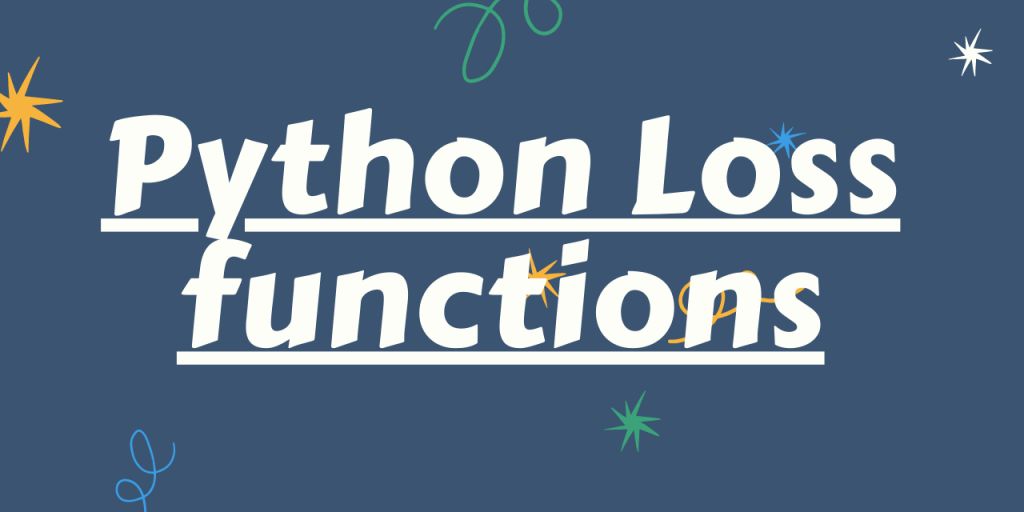# Overview of Loss Functions in PythonSo, let us get started!! 🙂

## Importance of Python Loss functions

Python has consistently played an important role in the domain of data science and machine learning. When it comes to applying a model on a dataset, it is very important for us to understand its effect on the dataset in terms of accuracy and error rates. This helps us in understanding the effect of the model towards the dependent variable.

For the same, we have Loss functions offered by Python in place. With Loss functions, we can easily understand the difference between the predicted data values and the expected/actual data values. With these loss functions, we can easily fetch the error rate and hence estimate the accuracy of the model based on it.

## 4 Mostly used Python Loss Functions

Having understood about Loss functions in Python, now we will be having a look at some of the mostly used Loss functions for error estimation and accuracy rate.

1. Root Mean Square Error
2. Mean Absolute Error
3. Cross Entropy function
4. Mean Square Error

### 1. Root Mean Square Error

With Root Mean Square Error, we calculate the difference between the predicted and the actual values of the dataset. Further, we calculate the square of the differences and then apply the mean function to it. Here, will be making use of the NumPy module and mean_squared_error() function altogether as shown below. With the mean_squared_error() function, we need to set the squared parameter to False, for it to pick up and calculate RMSE. If set to True, it will calculate MSE.

Example:

```from sklearn.metrics import mean_squared_error
import numpy as np
ac = np.array([1,2,3])
pr = np.array([0.9,1.9,2.1])
print(mean_squared_error(ac, pr, squared = False))
```

Output:

```0.5259911279353167
```

### 2. Mean Absolute Error

Mean Absolute Error enables us to have the average of the absolute differences between the predicted and the actual data values of the dataset. Python offers us with mean_absolute_error() function to calculate the mean absolute error for any data range.

Example:

```from sklearn.metrics import mean_absolute_error
import numpy as np
ac = np.array([1,2,3])
pr = np.array([0.9,1.9,2.1])
print(mean_absolute_error(ac, pr))
```

Output:

```0.3666666666666667
```

### 3. Mean Square Error

After RMSE, Mean Square Error enables us to calculate the mean of the squared differences between the actual and the predicted data values at ease. We can make use of the mean_squared_error() function to calculate MSE for a data range as shown–

Example:

```from sklearn.metrics import mean_squared_error
import numpy as np
ac = np.array([1,2,3])
pr = np.array([0.9,1.9,2.1])
print(mean_squared_error(ac, pr, squared = True))
```

Output:

```0.2766666666666666
```

### 4. Cross-Entropy Loss function

RMSE, MSE, and MAE mostly serve for regression problems. The cross-entropy loss function is highly used for Classification type of problem statements. It enables us to define the error/loss rate for the classification type of problems against the categorical data variable.

The sklearn library of Python offers us with log_loss() function to handle and estimate the error rate for classification/categorical data variables.

Example:

```from sklearn.metrics import log_loss
op = log_loss(["Yes", "No", "No", "Yes","Yes","Yes"],[[10, 9], [39, 11], [8, 2], [35, 65], [12, 14], [12,12]])
print(op)
```

Output:

```0.6931471805599453
```

## Conclusion

By this, we have come to the end of this topic. Feel free to comment below, in case you come across any question.

For more such posts related to Python Programming, Stay tuned with us.

Till then, Happy Learning!! 🙂